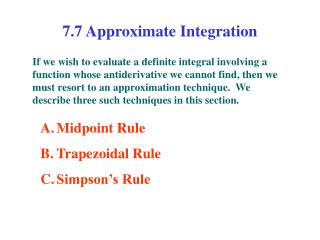DownloadDownload Presentation7.7 Approximate Integration

# 7.7 Approximate Integration

Télécharger la présentation## 7.7 Approximate Integration

- - - - - - - - - - - - - - - - - - - - - - - - - - - E N D - - - - - - - - - - - - - - - - - - - - - - - - - - -
##### Presentation Transcript

1. 7.7 Approximate Integration If we wish to evaluate a definite integral involving a function whose antiderivative we cannot find, then we must resort to an approximation technique. We describe three such techniques in this section. • Midpoint Rule • Trapezoidal Rule • Simpson’s Rule

2. y A. Midpoint Rule Let f be continuous on [a, b]. The Midpoint Rule for approximating is given by x a … b B. Trapezoidal Rule y Let f be continuous on [a, b]. The Trapezoidal Rule for approximating is given by x … C. Simpson’s Rule (n is even) y Let f be continuous on [a, b]. The Simpson’s Rule for approximating is given by x …

3. Example 1: Use (a) the Trapezoidal Rule, (b) the Midpoint Rule, and (c) the Simpson’s Rule to approximate the given integral with the specified value of n. (Round your answers to six decimal places.) (a) Trapezoidal Rule (b) Midpoint Rule (c) Simpson’s Rule

4. Example 2: The width (in meters) of a kidney-shaped swimming pool were measured at 2-meter intervals as indicated in the figure. Use Simpson’s Rule to estimate the area of the pool. 6.8 5.6 5.0 7.2 4.8 4.8 6.2 Solutions: Let x = distance from the left end of the pool w = w(x) = width at x